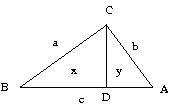My colleague Ricardo Pérez-Marco showed me a very cute proof of Pythagoras’ theorem, which I thought I would share here; it’s not particularly earth-shattering, but it is perhaps the most intuitive proof of the theorem that I have seen yet.In the above diagram, a, b, c are the lengths BC, CA, and AB of the right-angled triangle ACB, while x and y are the areas of the right-angled triangles CDB and ADC respectively. Thus the whole triangle ACB has area x+y.

Now observe that the right-angled triangles CDB, ADC, and ACB are all similar (because of all the common angles), and thus their areas are proportional to the square of their respective hypotenuses. In other words, (x,y,x+y) is proportional to$(a^2, b^2, c^2)$. Pythagoras’ theorem follows.

Here is a more “modern” way to look at Pythagoras’ theorem. The statement$a^2 + b^2 = c^2$ is equivalent to the assertion that the matrices$\begin{pmatrix} a & b \\ -b & a \end{pmatrix}$ and$\begin{pmatrix} c & 0 \\ 0 & c \end{pmatrix}$ have the same determinant. But it is easy to see geometrically that the linear transformations associated to these matrices differ by a rotation, and the claim follows.

Homework: why are the above two proofs essentially the same proof?